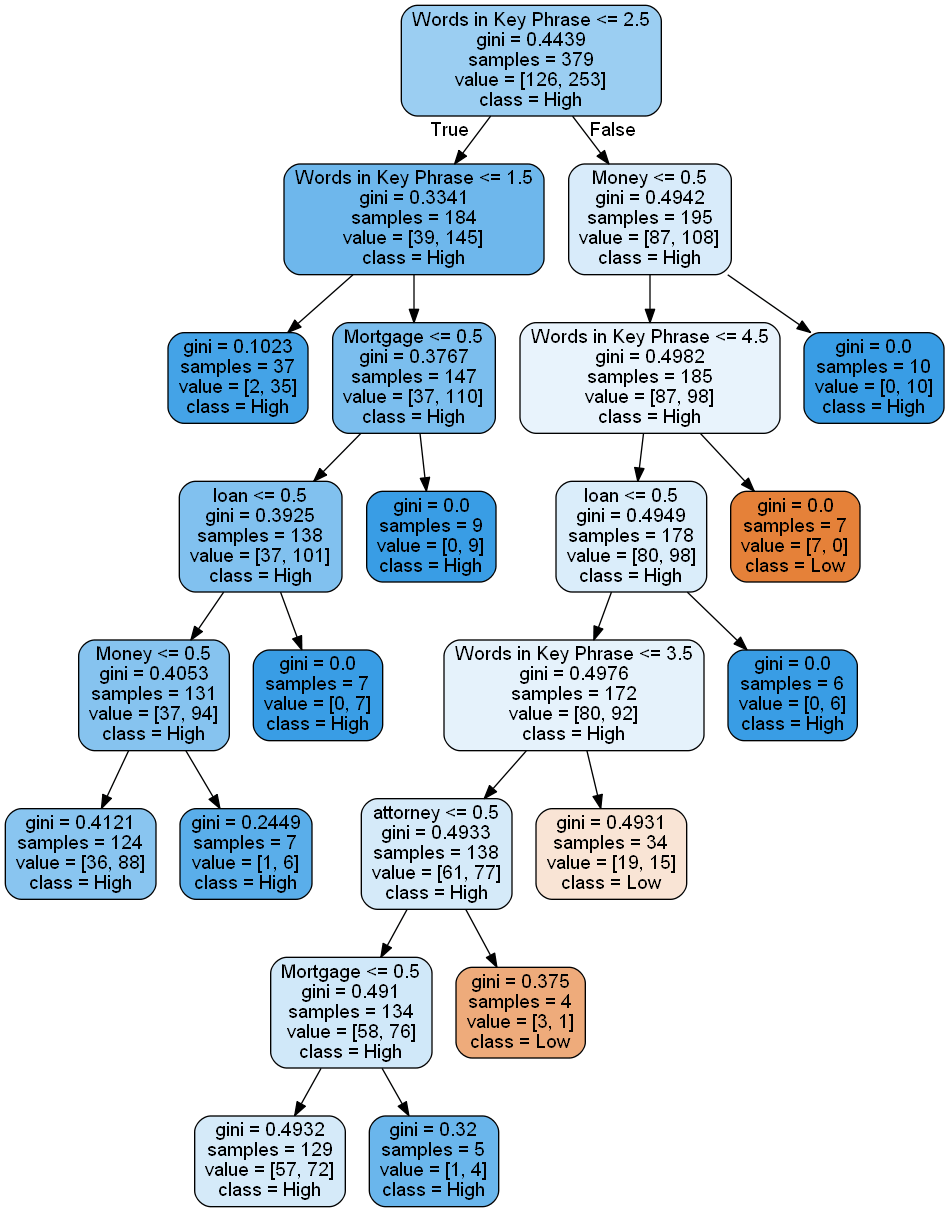# Building Decision Trees in Python – Handling Categorical Data

In the post Building Decision Trees in Python we looked at the decision tree with numerical continuous dependent variable. This type of decision trees can be called also regression tree.

But what if we need to use categorical dependent variable? It is still possible to create decision tree and in this post we will look how to create decision tree if dependent variable is categorical data. In this case the decision tree is called classification tree. Classification trees, as the name implies are used to separate the dataset into classes belonging to the response variable.  Classification is a typical problem that can be found in such fields as machine learning, predictive analytics, data mining.

Getting Data
For simplicity we will use the same dataset as before but will convert numerical target variable into categorical variable as we want build python code for decision tree with categorical dependent variable.
To convert dependent variable into categorical we use the following simple rule:
if CPC < 22 then CPC = "Low"
else CPC = “High”

For independent variables we will use “keyword” and “number of words” fields.
Keyword (usually it is several words) is a categorical variable. In general it is not the problem as in either case (regression or classification tree), the predictors or independent variables may be categorical or numeric. It is the target variable that determines the type of decision tree needed.

However sklearn.tree (at least the version that is used here) does not support categorical independent variable. See discussion and suggestions on stack overflow . To use independent categorical variable, we will code the categorical feature into multiple binary features. For example, we might code [‘red’,’green’,’blue’] with 3 columns, one for each category, having 1 when the category match and 0 otherwise. This is called one-hot-encoding, binary encoding, one-of-k-encoding or whatever. 

Below are shown few rows from the table data. We added 2 columns for categories “insurance”, “Adsense”. We actually have more categories and therefore we added more columns but this is not shown in the table.

For small dataset such conversion can be done manually. But we also created python script in the post Converting Categorical Text Variable into Binary Variables for this specific task. 

 Keyword Number of words Insurance Adsense CTR Cost Cost (categorical) car insurance premium 3 1 0 0.012 20 Low AdSense data 2 0 1 0.025 1061 High

Building the Code
Now we need to build the code. The call for decision tree is looking like this:

clf_gini = DecisionTreeClassifier(criterion = “gini”, random_state = 100,
max_depth=8, min_samples_leaf=4)

we use here criterion Gini index for splitting data.
In the call to export_graphviz we specify class names:

export_graphviz(tree, out_file=f, feature_names=feature_names, filled=True, rounded=True, class_names=[“Low”, “High”] )

The rest of the code is the same as in previous post for regression tree.

Here is the python computer code:


# -*- coding: utf-8 -*-

import pandas as pd
from sklearn.cross_validation import train_test_split
from sklearn.tree import DecisionTreeClassifier
import subprocess

from sklearn.tree import  export_graphviz

def visualize_tree(tree, feature_names):

with open("dt.dot", 'w') as f:

export_graphviz(tree, out_file=f, feature_names=feature_names,  filled=True, rounded=True, class_names=["Low", "High"] )

command = ["C:\\Program Files (x86)\\Graphviz2.38\\bin\\dot.exe", "-Tpng", "C:\\Users\\Owner\\Desktop\\A\\Python_2016_A\\dt.dot", "-o", "dt.png"]

try:
subprocess.check_call(command)
except:
exit("Could not run dot, ie graphviz, to "
"produce visualization")

data = pd.read_csv('adwords_data.csv', sep= ',' , header = 1)

X = data.values[:, [3,17,18,19,20,21,22]]
Y = data.values[:,8]

X_train, X_test, y_train, y_test = train_test_split( X, Y, test_size = 0.3, random_state = 100)

clf_gini = DecisionTreeClassifier(criterion = "gini", random_state = 100,
max_depth=8, min_samples_leaf=4)
clf_gini.fit(X_train, y_train)

visualize_tree(clf_gini, ["Words in Key Phrase", "AdSense",	"Mortgage",	"Money",	"loan", 	"lawyer", 	"attorney"])Decision Tree (partial view)

### 1 thought on “Building Decision Trees in Python – Handling Categorical Data”

1.owygs156

I created new post regression-and-classification-decision-trees-building-with-python with even more simplified conversion of categorical variables. In this post I am using built in python method to convert data.